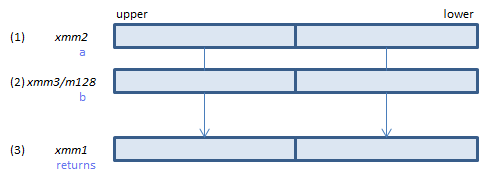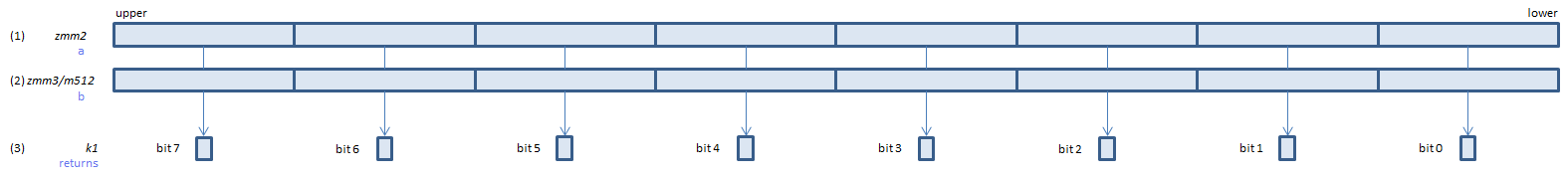﻿ vcmptruepd

## VCMPTRUEPD - CoMPare TRUE Packed Double

VCMPTRUEPD xmm1, xmm2, xmm3/m128    (V1
__m128d _mm_cmp_pd(__m128d a, __m128d b, _CMP_TRUE_UQ)For each double, always set 1 to all bits of the corresponding double of (3).
VCMPTRUEPD ymm1, ymm2, ymm3/m256    (V1
__m256d _mm256_cmp_pd(__m256d a, __m256d b, _CMP_TRUE_UQ)For each double, always set 1 to all bits of the corresponding double of (3).
VCMPTRUEPD k1{k2}, xmm2, xmm3/m128/m64bcst    (V5+VLFor each double, always set 1 to the corresponding bit of (3).
If k2 bit is 0, the comparison is not done and the corresponding bit of (3) is set to zero. Upper bits of (3) are zero cleared.
VCMPTRUEPD k1{k2}, ymm2, ymm3/m256/m64bcst    (V5+VLFor each double, always set 1 to the corresponding bit of (3).
If k2 bit is 0, the comparison is not done and the corresponding bit of (3) is set to zero. Upper bits of (3) are zero cleared.
VCMPTRUEPD k1{k2}, zmm2, zmm3/m512/m64bcst{sae}    (V5